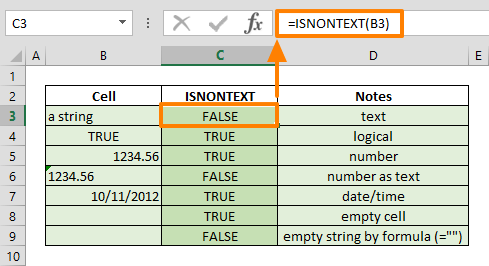The Excel ISNONTEXT function is an Information formula that returns TRUE if the input value is a not text value, and FALSE otherwise. You can test the result of a formula or value of a cell using this function. This formula can be especially useful in organizing data, troubleshooting, and data validation. In this guide, we’re going to show you how to use the Excel ISNONTEXT function and also go over some tips and error handling methods.

## Supported versions

• All Excel versions

ISNONTEXT(value)

## Arguments

 value The value or reference you want to test.

## Excel ISNONTEXT Function Sample Use Case

The ISNONTEXT function, just like other IS… functions, only requires a single argument as an input. A common use case for this function is testing the value of a cell or a value that is returned by a formula. The function returns a TRUE logical value, if the value argument is a non-text value. such as a number, date, error message string, or logical value.

=ISNONTEXT(100) returns TRUE

=ISNONTEXT("a string") returns FALSE## Tips

• You can use the ISNONTEXT function as a logical test inside an IF function.
=IF(ISNONTEXT(A1),"Non-text","Text")
• The function returns TRUE for empty cells. On the other hand, if a formula returns an empty string (""), the cell will look empty in the Excel interface, but the ISNONTEXT function will return FALSE in this case, as Excel considers the space character to be a non-empty value.
• IFERROR function can return a specific value if case of an error, and can be useful for error handling.
• Below is a list of other IS… functions:
• ISBLANK to test blank cells
• ISERR to test error values except #N/A
• ISERROR to test all error values
• ISNA to test #N/A error value specifically
• ISNUMBER to test numbers
• ISTEXT to test any item that is not a text
• ISREF to test a reference
• ISEVEN to test even numbers
• ISODD to test odd numbers